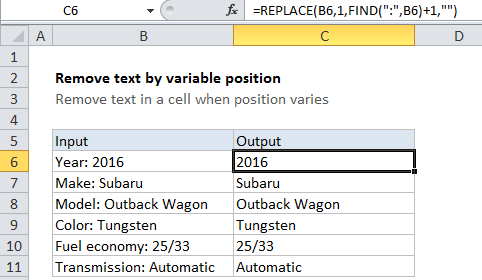# Remove text by variable position in a cell in Excel

To remove text from a cell when the text is at a variable position, you can use a formula based on the REPLACE function, with help from the FIND function.

## Formula

`=REPLACE(text,start,FIND(marker,text)+1,"")`## Explanation

In the example shown, the formula in C6 is:

`=REPLACE(B6,1,FIND(":",B6)+1,"")`

### How this formula works

The REPLACE function will replace text by position. You can use REPLACE to remove text by providing an empty string (“”) for the “new_text” argument.

Worked Example:   Split dimensions into three parts in Excel

In this case, we want to remove the labels that appear inside text. The labels vary in length, and include words like “Make”, “Model”, “Fuel economy”, and so on. Each label is followed by a colon and a space. We can use the colon as a “marker” to figure out where the label ends.

Worked Example:   Highlight cells that begin with in Excel

Working from the inside out, we use the FIND function to get the position of the colon in the text, then add 1 to take into account the space that follows the colon. The result (a number) is plugged into the REPLACE function for the “num_chars” argument, which represents the number of characters to replace.

Worked Example:   Split text and numbers in Excel

The REPLACE function then replaces the text from 1 to “colon + 1” with “”. In the example shown, the solution looks like this:

```=REPLACE(B6,1,FIND(":",B6)+1,"")
=REPLACE(B6,1,6,"")
=2016```